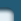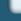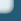Delay Equations

Web design by Alexei Kuntsevich## Selected Publications

### Keyword(s): delay equations


F. DiPasquantonio and F. Kappel. Applications to nuclear reactor kinetics of an extension of Liapunov's direct method to functional-differential equations. Energia Nucleare, 15:761–770, 1968.


F. Kappel. The invariance of limit sets for autonomous functional-differential equations. SIAM J. Appl. Math., 19:408–420, 1970.


F. Kappel. An invariance principle for functional-differential equations of retarded type. In Symposium on Differential-Delay and Functional Equations: Control and Stability, Warwick, June 26–July 7, 1972, volume 12 of Control Theory Centre Reports, pages 37–39. University of Warwick, 1972.


F. Kappel. Differenzen-Differentialgleichungen. In Proc. 5. Steiermärkisches Mathematisches Symposium, Stift Rein, September 24–27, 1973, number 1/1973 in Berichte der Mathematisch-Statistischen Sektion, Research Center Graz, pages 1–77, 1973.


F. Kappel. Some remarks to the problem of degeneracy for functional-differential equations. In Equa-Diff 73, Bruxelles et Louvaine-la-Neuve, September 3–8, 1973, pages 463–471, 1973.


F. Kappel. Degeneracy of functional-differential equations. In H. A. Antosiewics, editor, International Conference on Differential Equations, Proc. Internatl. Symp. Diff. Eqs., Los Angeles, September 3–7, 1974, pages 434–448, New York, 1975. Academic Press.


F. Kappel and H. K. Wimmer. An elementary divisor theory for linear autonomous functional differential equations. J. Differential Eqs., 21:134–147, 1976.


F. Kappel. Laplace-transform methods and linear autonomous functional-differential equations. Ber. Math.-Statist. Sekt. 64, Research Center Graz, 1976.


F. Kappel. On degeneracy of functional-differential equations. J. Differential Eqs., 22:250–267, 1976.


F. Kappel. A stability criterion for linear autonomous functional differential equations. In L. Cesari, J. K. Hale, and J. P. LaSalle, editors, Dynamical Systems, An International Symposium, Proc. Internatl. Symp. Dynamical Systems, Providence, August 12–16, 1974, pages 103–107, New York, 1976. Academic Press.


F. Kappel. Degenerate difference-differential equations: Algebraic theory. J. Differential Eqs., 24:99–126, 1977.


F. Kappel and W. Schappacher. Approximation of functional differential equations by finite dimensional systems. In R. Conti, G. Sestini, and G. Villari, editors, Equazioni differenziali ordinarie ed equazioni funzionali, Proc. Equa-Diff 78, Florence, May 24–30, 1978, pages 479–484, 1978.


F. Kappel and W. Schappacher. Autonomous nonlinear functional differential equations and averaging approximations. Nonlinear Analysis: TMA, 2:391–422, 1978.


F. Kappel. Approximation of functional-differential equations by ordinary differential equations and hereditary control problems. In J. Stoer, editor, Optimization Techniques, Vol. I, Proc. 8th IFIP Conf. Optimization Techniques, Würzburg, September 5–9, 1977, volume 6 of Lecture Notes in Control and Information Sciences, pages 103–108, Berlin, 1978. Springer Verlag.


H. T. Banks and F. Kappel. Spline approximations for functional differential equations. J. Differential Eqs., 34:496–522, 1979.


F. Kappel and W. Schappacher. Nonlinear functional differential equations and abstract integral equations. Proc. Royal Soc. Edinburgh, 84A:71–91, 1979.


F. Kappel and W. Schappacher. Some considerations to the fundamental theory of infinite delay equations. J. Differential Eqs., 37:141–183, 1980.


F. Kappel and K. Kunisch. Spline approximations for neutral functional differential equations. SIAM J. Numerical Analysis, 18:1058–1080, 1981.


F. Kappel. Approximation of neutral functional differential equations in the state space Rn times Lp. In M. Farkas, editor, Qualitative Theory of Differential Equations, Vol. I, Colloquia Mathematica Societatis Janos Bolyai, Vol. 30, pages 463–506. Janos Bolyai Math. Soc. and North Holland Publ. Comp., 1982.


F. Kappel. An approximation scheme for delay equations. In V. Lakshmikantham, editor, Nonlinear Phenomena in Mathematical Sciences, Proc. Internat. Conf. Nonl. Phenomena Math. Sci., Arlington (Texas), June 16–20, 1980, pages 585–595, New York, 1982. Academic Press.


F. Kappel and K. Kunisch. Approximation of the state of infinite delay and Volterra-type equations. In L. Collatz, G. Meinardus, and W. Wetterling, editors, Differenzen-Differentialgleichungen, Anwendungen und numerische Probleme, Proc. Workshop in Oberwolfach, June 6–12, 1982, pages 149–168, Basel, 1983. Birkhäuser.


F. Kappel, K. Kunisch, and G. Moyschewitz. An approximation scheme for infinite delay equations of Volterra type: Numerical results. Technical Report 11, Institutes for Mathematics–Technical University and University of Graz, 1983.


F. Kappel. Finite dimensional approximation to systems with infinite dimensional state space. In H.-W. Knobloch and K. Schmitt, editors, Equadiff 82, Proc. Equa-Diff 82, Würzburg, August 23–28, 1982, volume 1017, pages 287–299, Berlin, 1983. Springer Verlag.


F. Kappel and R. Grimmer. Series expansions for resolvents of Volterra integrodifferential equations in Banach spaces. SIAM J. Math. Analysis, 15:595–604, 1984.


F. Kappel and G. Propst. Approximation of feedback controls for delay systems using Legendre polynomials. Confer. Mat. Sem. Mat. Univ. Bari, 201:1–36, 1984.


F. Kappel, K. Kunisch, and G. Moyschewitz. An approximation scheme for parameter estimation in infinite delay equations of Volterra type: Numerical results. Technical Report 51, Institutes for Mathematics – Technical University and University of Graz, 1984.


F. Kappel. Linear autonomous functional differential equations in the state space C. Technical Report 34, Institutes for Mathematics–Technical University and University of Graz, 1984.


F. Kappel. Galerkin type approximation schemes for delay systems. Annales of Differential Equations, 1:57–82., 1985.


F. Kappel and Kangpei Zhang. Equivalence of functional-differential equations of neutral type and abstract Cauchy problems. Monatshefte für Mathematik, 101:115–133, 1986.


F. Kappel and Kangpei Zhang. On neutral functional differential equations with nonatomic D-operator. J. Math. Analysis Appl., 113:311–343, 1986.


F. Kappel. Semigroups and delay equations. In H. Brezis, M. G. Crandall, and F. Kappel, editors, Semigroups, Theory and Applications, Vol II, volume 152, pages 136–176, Harlow, 1986. Longman.


F. Kappel. Spline approximation for autonomous nonlinear functional differential equations. Nonlinear Analysis: TMA, 10:503–513, 1986.


F. Kappel and K. Kunisch. Invariance results for delay and Volterra equations in fractional order Sobolev spaces. Trans. AMS, 304:1–51, 1987.


F. Kappel and D. Salamon. Spline approximation for retarded systems and the Riccati equation. SIAM J. Control and Optimization, 25:1082–1117, 1987.


F. Kappel and K. Schmitt. Periodic solutions of systems of ordinary differential equations which approximate delay equations. Differential and Integral Eqs., 1:183–212, 1988.


K. Ito and F. Kappel. Approximation of infinite delay and Volterra type equations. Numerische Math., 54:405–444, 1989.


F. Kappel and G. Peichl. Preservation of controllability under approximation for delay systems. Matematica Aplicada e Computational, 8:23–47, 1989.

This paper deals with controllability of differential equations with a single delay and its finite dimensional approximations. A set of hypotheses is presented which allow a unified treatment of various approximation schemes. Controllability of the delay equation as well as of the approximating ordinary differential equations is characterized by a finite number of linear homogeneous equations which in both cases are closely related. For the approximating systems the size of the linear system of equations which characterizes controllability is independent of the order of approximation.


F. Kappel and D. Salamon. On the stability properties of spline approximation for retarded systems. SIAM J. Control and Optimization, 27:407–431, 1989.


F. Kappel and D. Salamon. An approximation theorem for the algebraic Riccati equation. SIAM J. Control and Optimization, 28:1136–1147, 1990.

For an infinite dimensional linear quadratic control problem in Hilbert space, approximation of the solution of the algebraic Riccati operator equation in the strong operator topology is considered under conditions weaker than uniform exponential stability of the approximating systems. As an application strong convergence of the approximating Riccati operators in case of a previously developed spline approximation scheme for delay systems is established. Finally we investigate convergence of the transfer-functions of the approximating systems.


K. Ito and F. Kappel. On integro-differential equations with weakly singular kernels. In J. Goldstein, F. Kappel, and W. Schappacher, editors, Differential Equations with Applications in Biology, Physics and Engineering, pages 209–218, New York, 1991. Marcel-Dekker.

In this paper we consider a class of integro-differential equations with weakly singular kernels. Such an equation is treated using the theory of linear C0-semigroups. Differentiability of the solution semigroup and well-posedness in case of non-integrable kernels on (- infty ,0) are established.


K. Ito and F. Kappel. A uniformly differentiable approximation scheme for delay systems using splines. Appl. Math. Optim., 23:217–262, 1991.

A new spline-based scheme is developed for linear retarded functional differential equations within the framework of semigroups on the Hilbert space bold Rn times L2. The approximating semigroups inherit in a uniform way the characterization for differentiable semigroups from the solution semigroup of the delay system (e.g. among other things the logarithmic sectorial property for the spectrum). We prove convergence of the scheme in the state spaces bold Rn times L2 and H1. The uniform differentiability of the approximating semigroups enables us to establish error estimates including quadratic convergence for certain classes of initial data. We also apply the scheme for computing the feedback solutions to linear quadratic optimal control problems.


K. Ito, F. Kappel, and D. Salamon. A variational approach to approximation of delay systems. Differential and Integral Eqs., 4:51–72, 1991.

Using a varational formulation of the Trotter-Kato approximation theorem for strongly continuous semigroups we show that one can construct in a uniform way most of the approximation schemes for delay systems which have been published in recent years. In order to demonstrate that this approach is not restricted to delay systems we also construct two approximation schemes for size structured population models.


F. Kappel. Approximation of LQR-problems for delay systems: a survey. In K. Bowers and J. Lund, editors, Computation and Control II, pages 187–224, Boston, 1991. Birkhäuser.


K. Ito and F. Kappel. Two families of approximation schemes for delay systems. Results in Mathematics, 21:93–137, 1992.

Based on the Trotter-Kato approximation theorem for strongly continuous semigroups we develop a general framework for the approximation of delay systems. Using this general framework we construct two families of concrete approximation schemes. Approximation of the state is done by functions which are piecewise polynomials on a mesh (m-th order splines of deficiency m). For the two families we also prove convergence of the adjoint semigroups and uniform exponential stability, properties which are essential for approximation of linear quadratic control problems involving delay systems. The characteristic matrix of the delay system is in both cases approximated by matrices of the same structure but with the exponential function replaced by approximations where Padé fractions in the main diagonal resp. in the diagonal below the main diagonal of the Padé table for the exponential function play an essential role.


F. Kappel and J. Turi. Singular integro-differential equations and product spaces. J. Math. Analysis Appl., 178:450–469, 1993.

For certain types of integro-differential equations with weakly singular kernels we investigate well-posedness in a state space with product space structure. This state space is obtained as the extrapolation space corresponding to the solution semigroup of the problem on a weighted L2-space, where well-posedness has been established recently.


F. Kappel. Parameteridentification for state dependent delays originating from threshold conditions. In Proc. IEEE Mediterranean Symposium on New Directions in Control Theory and Applications, June 21 - 23, 1993, Crete Chandris Hotel, Maleme, Crete, Chania, 1993. Technical University of Crete.


K. Ito, F. Kappel, and J. Turi. On well-posedness of singular neutral equations in the state space C. J. Differential Eqs., 125:40–72, 1996.

In this paper we study necessary und sufficient conditions for well-posedness of scalar, singular (i.e., with a difference operator which is nonatomic at zero) neutral functional differential equations (SNFDEs) in the state space C . We also discuss an ill-posed SNFDE in order to illustrate how the addition of a singularity away from zero (in our case we have a perturbing atom at -r) can destroy well-posedness.


F. Kappel, V. I. Maksimov, and E. N. Skuratov. On dynamical reconstruction of control in a system with time delay: Finite-dimensional models. In I. I. Eremin, I. Lasicka, and V. I. Maksimov, editors, Distributed Systems: Optimization and Economic-Environmental Applications (DSO'2000), Proceedings of an Internatl. Conf., May 30 – June 2, 2000, Ekaterinburg, Russia, pages 262–264, 2000. ISBN 5–7691–1047–3.

The problem of dynamical reconstruction of an unknown control acting upon a linear system with time delay through inaccurate observation of phase states is discussed. Regularized solving algorithm functioning in real time mode is proposed. This algorithm is stable with respect to informational noises and computational errors.


K. Ito and F. Kappel. Locally quasi-dissipative evolution equations and applications to delay equations. submitted.

In this paper we discuss applications of the generation theory of nonlinear semigroups for evolution equations with locally quasi-dissipative operators in the sense of Kobayashi and Oharu to delay differential equations.